## research papersJOURNAL OFAPPLIEDCRYSTALLOGRAPHY
ISSN: 1600-5767
Volume 37| Part 4| August 2004| Pages 545-550

## CRYSTALS enhancements: refinement of atoms continuously disordered along a line, on a ring or on the surface of a sphere

aLaboratoire Léon Brillouin (CEA–CNRS), CE Saclay – Bâtiment 563, 91191 Gif-Sur-Yvette Cedex, France, bChemical Crystallography, Chemistry Research Laboratory, University of Oxford, Mansfield Road, Oxford OX1 3TA, UK, and cRWTH Aachen, Institut für Kristallographie, Jägerstraße 17–19, 52056 Aachen, Germany
*Correspondence e-mail: david.watkin@chem.ox.ac.uk

(Received 16 March 2004; accepted 22 April 2004)

The program CRYSTALS [Betteridge, Carruthers, Cooper, Prout & Watkin (2003). J. Appl. Cryst. 36, 1487] has been extended to include an option for the refinement of a continuous electron density distribution lying along a line, a ring or on the surface of a sphere. These additional non-atomic electron distributions can be refined in any combination with traditional anisotropically distributed spherical atoms, including the refinement of partial' atoms overlapping with the special electron distributions.

### 1. Introduction

The crystallographic literature contains many examples of cases where good computational ideas have been incorporated into unsupported software, so that working versions of the programs soon become impossible to find. Amongst the aims of the monolithic program system CRYSTALS (Betteridge et al. 2003) have been the desires both to develop new ideas and strategies, and to incorporate into a stable platform good ideas taken from elsewhere. We describe here the reimplementation of an idea initially developed outside of Oxford.

Most of the widely distributed programs for single-crystal X-ray structure analysis only permit the structure to be described in terms of atoms with spherical form factors and isotropic or second-order tensor anisotropic atomic displacement parameters (a.d.p.'s). These parameterizations of electron distribution can be used to model disorder, but this process requires the introduction of partial' atoms distributed throughout the diffuse electron density and organized so that there is a sensible relationship between their a.d.p.'s; the total site occupancy of these atoms is also controlled. Some programs give the user a warning if the a.d.p.'s of single atoms become sufficiently anisotropic to justify the introduction of split' atoms, i.e. the distributed density can perhaps be better represented by two atoms. The a.d.p.'s and molecular geometry of these split atoms may have to be controlled by restraints or constraints. The same situation can arise in neutron diffraction, where the nuclear density is distributed over a site larger than that normally ascribed to an ordered atom. In this paper density' will refer to either nuclear or electron density, and form factor' will refer either to atomic form factor or to nuclear scattering length. If more than two atoms become necessary to model the disorder, smeared out' electron density may provide an alternative model.

Programs have been designed and developed for local use (Chernyshev et al., 1992; Zlokazov & Chernyshev, 1992) which replace the point atom and its associated form factor and a.d.p.'s by a representation in which an atom or group of atoms are replaced by a simple geometrical shape. However, such programs are not widely available or supported for long. In the current development to CRYSTALS, the permitted shapes are a line segment, an annulus (ring) and a spherical shell (sphere). In each case, the density distribution of the shape is controlled by the form factor and an isotropic a.d.p. The length of the line and the radius of the annulus or shell are controlled by variable parameters, as is the location and orientation of the shape in the cell. The implementation permits atomic representations to coexist with the shape representations for the density, thus permitting non-uniform density distributions to be constructed.

### 2. Implementation considerations

Chernyshev et al. (1994) (CZYAS), besides giving a brief background to the problem, showed that it is possible to refine a structure model containing one or more of these special figures (line, ring, sphere) by introducing an additional factor into the calculation of the structure factors. The authors determined the appropriate contribution to the structure-factor expression for electron density distributed on the surface of a sphere, a line and a ring (Appendix A).

Inclusion of these special shapes in a refinement program can be seen as equivalent to introducing into the structure-factor expression more complicated (and possibly spatially directed) modifications to the conventional a.d.p. terms. The sphere (hollow shell) is characterized by six parameters, namely a refinable centre of action (x), an effective radius, a site-occupancy factor and an isotropic displacement parameter (d.p.). The line is characterized by x, an occupancy, an isotropic d.p., a length' for the line segment and parameters giving the orientation of the line in the cell. The annulus is characterized by x, an occupancy, an isotropic d.p., an effective radius and parameters giving the orientation of the ring in the cell. CZYASmodel the density distribution for the ring by a zero-order Bessel function. Computational difficulties with single-precision (32 bit) arithmetic led us to use two approximations instead, described by Press et al. (1986).

The line and ring are orientated shapes, requiring refinable parameters to describe the orientation. These parameters eventually appear in the CZYAScalculations as parameter ψ, which is the angle between the scattering vector and the line or ring normal. The propagation of ψ into the structure-factor expression is best developed in terms of the triclinic unit vector representing the line or ring normal. Only two of the three components are independent. This constraint is most easily handled by making the corresponding polar coordinates of the unit vector the actual least-squares variables and calculating ψ for each reflection via the orthogonal and then triclinic components in real space, followed by a transformation into reciprocal space (Boisen & Gibbs, 1990). The polar coordinates are an angle of declination [angle between the z axis of a Cartesian system (Rollett, 1965) and the unit vector] and an angle of azimuth (angle between the projection of the unit vector onto the plane containing the x and y axis of the Cartesian system and the x axis). This representation, with the angles given in degrees, is probably also convenient for the program user. Fully expanded, the elegant equations of CZYASbecome less attractive; these are given explicitly in Appendix A1. The derivatives are given in Appendix A2, and the meaning of symbols in Appendix A3.

#### 2.1. Implementation details

The singularities latent in the expanded equations were investigated numerically, and it was found that all the limiting cases were finite and so could be accommodated in the final program. However, providing a mathematical solution to a problem is not sufficient if a program is to find wide acceptance. Users of the program must be provided with a reasonably easy-to-use interface to the mathematics. All of the existing program structure has been preserved and extended in a consistent manner to include these new variables. It is therefore possible to refine structures containing both normal atoms and these special figures. It is even possible to embed atoms in the special figures and set relationships between their site-occupation factors. The modelling of distributed density containing local maxima is hence permitted, thus providing a partial solution to the problem of modelling a hindered rotator' (King & Lipscomb, 1950; Bennett et al., 1975), pending a full reimplementation of the algorithms of these authors. Fig. 1is a screen capture showing how annuli with embedded partial atoms appear in the CRYSTALS model window. The inner ring of atoms is isotropic, the outer anisotropic. An alternative approach to modelling via the discrete Fourier transform of the difference density (Spek, 1994) is often more suitable for static disorder.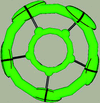Figure 1 CRYSTALS screen capture of a Cp* fragment consisting of partial atoms embedded in annuli.

Tools are included for converting groups of normal atoms into the special figures. All the usual constraints and restraints available in the CRYSTALS program are available for the special figure parameters (e.g. they can be left unrefined, linked to other parameters or given soft values via restraints). Symmetry restrictions for a special figure on a special position are not yet worked out automatically by CRYSTALS, so that the user must specify them explicitly. For the monoclinic system, this calculation is simplified by using the second setting, which makes the unique axis parallel to z of the Cartesian system so that the angles of declination and azimuth are evident. For example, an annulus lying perpendicular to the c axis would have the angles of declination and azimuth constrained to zero.

#### 2.2. Validation

It is extremely difficult to prove that code of this complexity is working correctly, though various tests may suggest its validity. One test is to check the self-consistency by creating a special figure and generating a set of structure factors from this model to be used as observations' in a subsequent refinement. The model used to generate the structure factors should remain unchanged after least squares. As a further test, the model can be perturbed before refinement. It should return to the starting value after least squares. It was found that the range of convergence was similar to that of conventional least squares.

An indication of the physical reasonableness of the structure factors generated by the algorithm can be obtained by plotting out their Fourier transform. A structure containing pentamethylcyclopentene was modified so that only the ten Cp* atoms remained. Structure factors were generated from this model and used as pseudo-observations, Fo'. The inner and outer rings of C atoms were alternately replaced by ring' special figures. The special-figure parameters (centroid, radius, Uiso, declination and azimuth) were refined to convergence, and structure factors were computed (giving Fcalc and α). The Fourier transforms are shown in the accompanying figures. Fig. 2shows the electron density computed from the Fobs' and α computed from a normal atomic model. Fig. 3shows density computed from the Fobs' and phases computed from a ring figure used in place of the methyl groups. There is more or less continuous density, with only slight peaks at the original atom sites. This map is a striking example of how the phases dominate the features of Fourier syntheses. Fig. 4is a similar map, using both structure factors and phases computed from the ring special shape, and shows that the Fourier transform of the structure factors generated for the special shape is indeed an annulus. Fig. 5is a section perpendicular to Fig. 4, passing through one inner C atom (left of centre) and between two atoms (right of centre). The outer low peaks are sections through the annulus. Figs. 6and 7correspond to Figs. 3and 4but with the inner ring of atoms replaced by the special figure. Table 1lists some properties of the original ten-atom fragment and the special-figure parameters. Note that the difference in the radii of the two rings corresponds closely to a CCp—Cmethyl bond length.

 Table 1Comparison of the atomic model and the annular model parameters
 Inner refers to the annular figure replacing the cyclopentadiene atoms and outer refers to the annular figure replacing the methyl atoms.
 Ideal CCp—Cmethyl bond length 1.49 Å Radiusouter − Radiusinner 1.48 Å Mean CCp Ueq 0.09 Å2 Inner Uiso 0.05 Å2 Mean CCp Umin 0.04 Å2 Mean CCp Umed 0.07 Å2 Mean CCp Umax 0.16 Å2 Mean Cmethyl Ueq 0.22 Å2 Outer Uiso 0.13 Å2 Mean Cmethyl Umin 0.05 Å2 Mean Cmethyl Umed 0.10 Å2 Mean Cmethyl Umax 0.51 Å2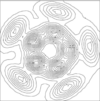Figure 2 Electron density based on structure factors computed from the distributed density and phases computed from an atomic model.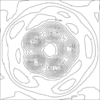Figure 3 Electron density based on structure factors computed from the distributed density and phases computed from a ring figure used in place of the methyl groups.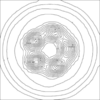Figure 4 Electron density based on both structure factors and phases computed from the ring special shape.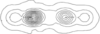Figure 5 Electron density based on a section perpendicular to Fig. 4, passing through one inner C atom (left of centre) and between two atoms (right of centre).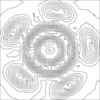Figure 6 This correspond to Fig. 3, but with the inner ring of atoms replaced by the special figure.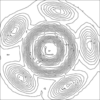Figure 7 This correspond to Fig. 4, but with the inner ring of atoms replaced by the special figure.

A number of structures have been refined using these distributed electron densities and have been published (Nicolaï et al., 2001, 2003; Fillaux et al., 2003).

#### 2.3. CIF data items

New CIF data items have been allocated to these electron distributions. The centroids, Uiso values and occupancies are associated with existing _atom_site_ data items, enabling existing plotting programs to at least indicate the centre of the shape. The additional items are given new names and can be included in a loop similar to the anisotropic a.d.p. loop.

### 3. Conclusions

The integration of these special electron distributions into CRYSTALS provides the user with considerable flexibility in modelling totally or partially distributed electron density. CRYSTALS normally takes care of atomic parameter restrictions for atoms in special positions. That part of the code has not yet been extended to cover the special figures. However, the user is always able to introduce restraints or constraints into the refinement manually, so that with thought, special figures can be refined even if they lie on sites of special symmetry. The ability to co-refine atomic sites and special figures, with relationships imposed to control total occupancy etc., provides a mechanism for modelling peaky' distributed density.

### 4. Availability

The CRYSTALS program for Windows is available free to not-for-profit organizations from the Oxford University Chemical Crystallography Laboratory website, http://www.xtl.ox.ac.uk .

### APPENDIX A

The equations given by CZYASbecome complicated when they are expanded into computable form in terms of the actual least-squares parameters. The forms we have used are listed in this appendix as an aid to other programmers.

The additional factors given by CZYAS:

1. Surface of a sphere: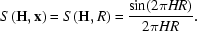2. Line: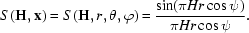3. Ring: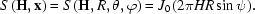#### A1. Additional factors in terms of the refinable parameters

1. Surface of a sphere:2. Line: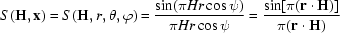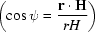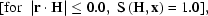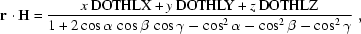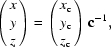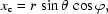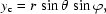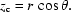3. Ring: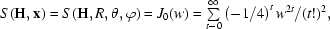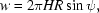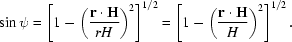Approximations: For w < 8.0,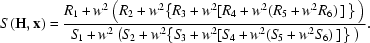For w > 8.0,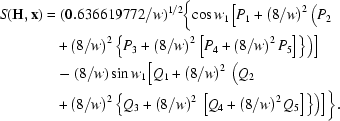#### A2. Derivatives

1. Surface of a sphere: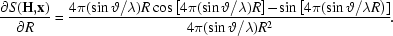2. Line: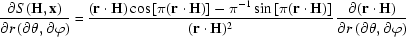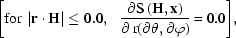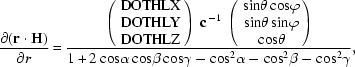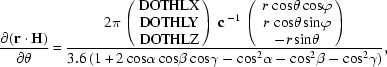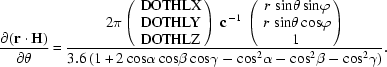3. Ring: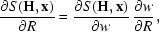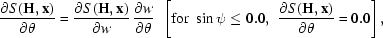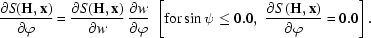For w ≤ 8.0,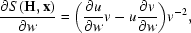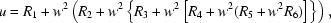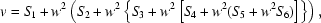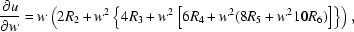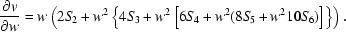For w > 8.0,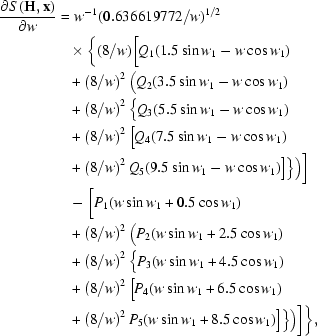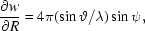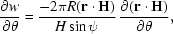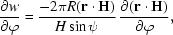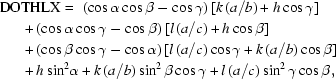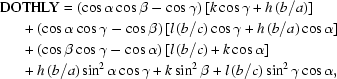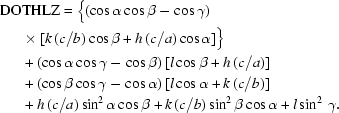#### A3. Abbreviations used

H: reflection vector in reciprocal space.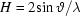: length of reflection vector.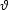: diffraction angle.

λ: wavelength.

x: parameters describing the sphere, the line or the ring.

R: radius of sphere or ring.

r: direction vector in reciprocal space.

r: length of direction vector (= 1 for the ring).

θ: declination angle describing the orientation of the direction vector.

φ: azimuthal angle describing the orientation of the direction vector.

ψ: angle between the direction vector in reciprocal space and H.

J0: Bessel function of 0th order (Press et al., 1986, p. 170).

w = 2πHRsinψ: argument of the Bessel function.

w1 = w - 0.785398164 (abbreviation).

R1R6, S1S6: constant parameters in the approximation Q1Q5, P1P5 of the Bessel function.

DOTHLX, DOTHLY, DOTHLZ: abbreviations.

x, y, z: triclinic coordinates.

xc, yc, zc: Cartesian coordinates.

c: orthogonalization matrix (Rollett, 1965).

a, b, c, α, β, γ: cell parameters.

h, k, l: reflection indices.

### Acknowledgements

LS expresses his gratitude and appreciation to different partners who ensured the financing and encouraged him in this work, particularly H. Noël (Rennes), F. Fillaux (LASIR, Thiais) and the CEA. The submitting author thanks the referees for their perceptive criticisms of the original manuscript.

### ReferencesJOURNAL OFAPPLIEDCRYSTALLOGRAPHY